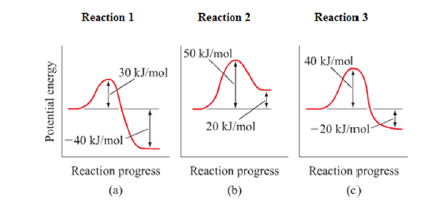# Problem: The potential energy profiles for three reactions are shown below.Which of the following is a  true statement?A) Endothermic rxns are always slower than exothermic rxnsB) The faster the rate of a reaction the more exothermic it must beC) Ea of rxn 1 is 2 times that of rxn 3D) For reaction 1, ΔHrxn = ‐70 k/molE) The Ea for the reverse reaction of rxn 2 is Ea2 = +30 kj/mol

🤓 Based on our data, we think this question is relevant for Professor Zaitoun's class at IVC.

###### Problem Details

The potential energy profiles for three reactions are shown below.

Which of the following is a  true statement?

A) Endothermic rxns are always slower than exothermic rxns
B) The faster the rate of a reaction the more exothermic it must be
C) Ea of rxn 1 is 2 times that of rxn 3
D) For reaction 1, ΔHrxn = ‐70 k/mol
E) The Ea for the reverse reaction of rxn 2 is Ea2 = +30 kj/molWhat scientific concept do you need to know in order to solve this problem?

Our tutors have indicated that to solve this problem you will need to apply the Energy Diagram concept. If you need more Energy Diagram practice, you can also practice Energy Diagram practice problems.

What is the difficulty of this problem?

Our tutors rated the difficulty ofThe potential energy profiles for three reactions are shown ...as high difficulty.

How long does this problem take to solve?

Our expert Chemistry tutor, Jules took 4 minutes and 57 seconds to solve this problem. You can follow their steps in the video explanation above.

What professor is this problem relevant for?

Based on our data, we think this problem is relevant for Professor Zaitoun's class at IVC.